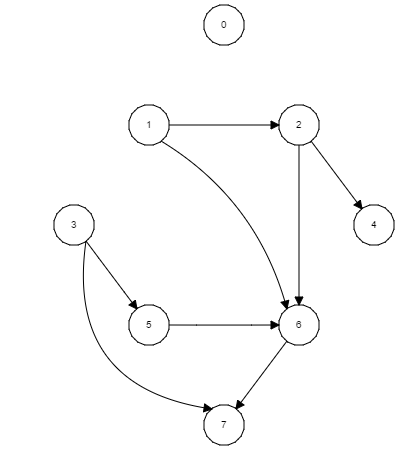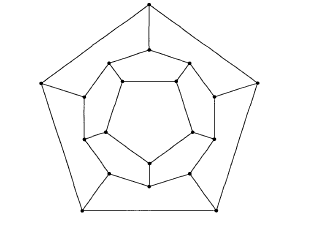Computer Science

# Graph implementation and representation

An Euler path in a graph is a path such that every edge is traversed exactly once. Consider the following adjacency matrix representation of the graph:

$A = \left(\begin{array}{lcccr} 0 & 2 & 1 & 0 & 0 \\ 2 & 0 & 2 & 0 & 0 \\ 1 & 2 & 0 & 2 & 2 \\ 0 & 0 & 2 & 0 & 2 \\ 0 & 0 & 2 & 2 & 0 \end{array}\right)$

Write pseudocode to check if the graph contains an Euler path.Consider the directed graph above. Which one of the following is a correct topological ordering of the graph?

A Hamilton path of a graph is a path that contains all vertices exactly once. If the last vertex in a Hamilton path has the first vertex as a neighbor, then the path can be turned into a Hamilton circuit by joining the last vertex in the path to the first vertex, i.e, a Hamilton path that is also a cycle is a Hamilton circuit.

Does the following graph allow a Hamilton circuit?In the adjacency matrix representation of the graph below, how many entries evaluate to True?Which of the following choices is the minimum spanning tree for the following weighted graph?ABCD×